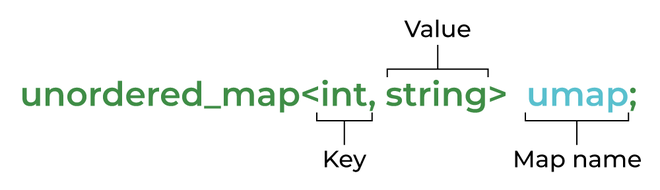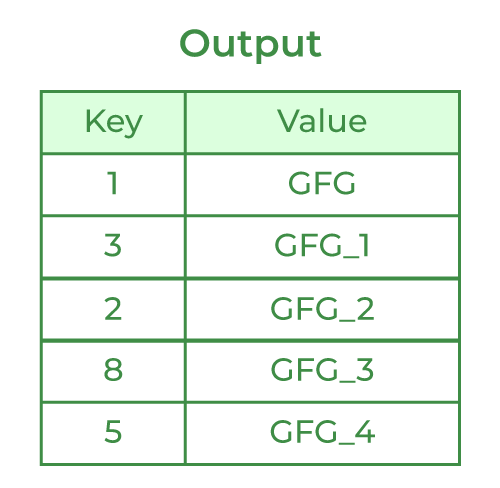Open in App
Not now

# unordered_map in C++ STL

• Difficulty Level : Easy
• Last Updated : 10 Jan, 2023

unordered_map is an associated container that stores elements formed by the combination of a key value and a mapped value. The key value is used to uniquely identify the element and the mapped value is the content associated with the key. Both key and value can be of any type predefined or user-defined. In simple terms, an unordered_map is like a data structure of dictionary type that stores elements in itself. It contains successive pairs (key, value), which allows fast retrieval of an individual element based on its unique key.

Internally unordered_map is implemented using Hash Table, the key provided to map is hashed into indices of a hash table which is why the performance of data structure depends on the hash function a lot but on average, the cost of search, insert, and delete from the hash table is O(1).

Note: In the worst case, its time complexity can go from O(1) to O(n), especially for big prime numbers. In this situation, it is highly advisable to use a map instead to avoid getting a TLE(Time Limit Exceeded) error.

Syntax:unordered_map syntax

Below is the C++ program to demonstrate an unordered map:

## C++

 `// C++ program to demonstrate ``// functionality of unordered_map``#include ``#include ``using` `namespace` `std;`` ` `// Driver code``int` `main()``{``  ``// Declaring umap to be of ``  ``// type key ``  ``// will be of STRING type ``  ``// and mapped VALUE will``  ``// be of int type``  ``unordered_map umap;`` ` `  ``// inserting values by using [] operator``  ``umap[``"GeeksforGeeks"``] = 10;``  ``umap[``"Practice"``] = 20;``  ``umap[``"Contribute"``] = 30;`` ` `  ``// Traversing an unordered map``  ``for` `(``auto` `x : umap)``    ``cout << x.first << ``" "` `<< ``            ``x.second << endl;``}`

Output

```Contribute 30
Practice 20
GeeksforGeeks 10
```unordered_map Output

Explanation: The specific thing that this output justifies is that the value of the outcome of unordered_map is produced in a random key-to-value manner whereas the map displays value and key in an ordered manner.

## unordered_map vs unordered_set

Note: For example, consider the problem of counting the frequencies of individual words. We can’t use unordered_set (or set) as we can’t store counts while we can use unordered_map.

## Methods on unordered_map

A lot of functions are available that work on unordered_map. The most useful of them are:

• operator =
• operator []
• empty
• size for capacity
• begin and end for the iterator.
• find and count for lookup.
• insert and erase for modification.

The below table shows the complete list of the methods of an unordered_map:

The C++11 library also provides functions to see internally used bucket count, bucket size, and also used hash function and various hash policies but they are less useful in real applications. We can iterate over all elements of unordered_map using Iterator.

## C++

 `// C++ program to demonstrate ``// Initialization, indexing, ``// and iteration``#include ``#include ``using` `namespace` `std;`` ` `// Driver code``int` `main()``{``  ``// Declaring umap to be of ``  ``// type key ``  ``// will be of string type and ``  ``// mapped value will be of double type``  ``unordered_map umap = {  ``//inserting element directly in map``  ``{``"One"``, 1},``  ``{``"Two"``, 2},``  ``{``"Three"``, 3}``};`` ` `  ``// inserting values by using [] operator``  ``umap[``"PI"``] = 3.14;``  ``umap[``"root2"``] = 1.414;``  ``umap[``"root3"``] = 1.732;``  ``umap[``"log10"``] = 2.302;``  ``umap[``"loge"``] = 1.0;`` ` `  ``// inserting value by insert function``  ``umap.insert(make_pair(``"e"``, 2.718));`` ` `  ``string key = ``"PI"``;`` ` `  ``// If key not found in map iterator ``  ``// to end is returned``  ``if` `(umap.find(key) == umap.end())``    ``cout << key << ``" not found\n\n"``;``   ` `  ``// If key found then iterator to that ``  ``// key is returned``  ``else``    ``cout << ``"Found "` `<< key << ``"\n\n"``;``   ` `  ``key = ``"lambda"``;``  ``if` `(umap.find(key) == umap.end())``    ``cout << key << ``" not found\n"``;``  ``else``    ``cout << ``"Found "` `<< key << endl;``   ` `  ``// iterating over all value of umap``  ``unordered_map::iterator itr;``  ``cout << ``"\nAll Elements : \n"``;``  ``for` `(itr = umap.begin(); ``       ``itr != umap.end(); itr++) ``  ``{``    ``// itr works as a pointer to ``    ``// pair type ``    ``// itr->first stores the key part and``    ``// itr->second stores the value part``    ``cout << itr->first << ``"  "` `<< ``            ``itr->second << endl;``  ``}``}`

Output

```Found PI

All Elements :
e  2.718
loge  1
log10  2.302
Two  2
One  1
Three  3
PI  3.14
root2  1.414
root3  1.732
```

### Find frequencies of individual words

Given a string of words, the task is to find the frequencies of the individual words:

Input: str = “geeks for geeks geeks quiz practice qa for”;
Output: Frequencies of individual words are
(practice, 1)
(for, 2)
(qa, 1)
(quiz, 1)
(geeks, 3)

Below is the C++ program to implement the above approach:

## C++

 `// C++ program to find freq ``// of every word using unordered_map``#include ``using` `namespace` `std;`` ` `// Prints frequencies of ``// individual words in str``void` `printFrequencies(``const` `string &str)``{``  ``// declaring map of type, ``  ``// each word is mapped to its frequency``  ``unordered_map wordFreq;``   ` `  ``// breaking input into word using ``  ``// string stream``  ``// Used for breaking words``  ``stringstream ss(str);``   ` `  ``// To store individual words``  ``string word; ``  ``while` `(ss >> word)``    ``wordFreq[word]++;`` ` `  ``// now iterating over word, freq pair ``  ``// and printing them in <, > format``  ``unordered_map:: iterator p;``  ``for` `(p = wordFreq.begin(); ``       ``p != wordFreq.end(); p++)``    ``cout << ``"("` `<< p->first << ``", "` `<<``                   ``p->second << ``")\n"``;``}`` ` `// Driver code``int` `main()``{``  ``string str = ``"geeks for geeks geeks quiz "``               ``"practice qa for"``;``  ``printFrequencies(str);``  ``return` `0;``}`

Output

```(practice, 1)
(for, 2)
(qa, 1)
(quiz, 1)
(geeks, 3)
```

Recent Articles on unordered_map